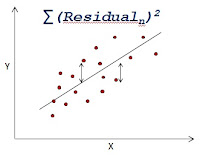## Pages

###Linear Regression - Connecting Dots

Linear regression analysis analyzes the linear relationship that exists between a Dependent Variable and Independent Variables. The Least squares criterion is used for determining a regression line that minimizes the sum of squared Residuals. For more watch this video tutorial. The video tutorial covers preparatory work required to be done before Linear Regression modeling.

Disclaimer : Video is linked to our YouTube channel earlier registered as simplified-SAS. Later we have renames it to Ask Analytics.

Linear Regression - Part 1 - Video Tutorial

Linear Regression - Part 2 - Video Tutorial